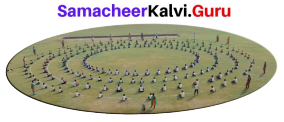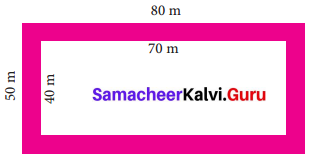# Samacheer Kalvi 7th Maths Solutions Term 2 Chapter 2 Measurements Ex 2.3

Students can Download Maths Chapter 2 Measurements Ex 2.3 Questions and Answers, Notes Pdf, Samacheer Kalvi 7th Maths Book Solutions Guide Pdf helps you to revise the complete Tamilnadu State Board New Syllabus and score more marks in your examinations.

## Tamilnadu Samacheer Kalvi 7th Maths Solutions Term 2 Chapter 2 Measurements Ex 2.3

Question 1.
Find the area of a circular pathway whose outer radius is 32 cm and inner radius is 18 cm.
Solution:
Radius of the outer circle R = 32 cm
Radius of the inner circle r = 18 cm
Area of the circular pathway = π (R2 – r2) sq. units = $$\frac { 22 }{ 7 }$$ (322 – 182) cm2
= $$\frac { 22 }{ 7 }$$ × (32 + 18) × (32 – 18) cm2
= $$\frac { 22 }{ 7 }$$ × 50 × 14 cm2 = 2,200 cm2
Area of the circular pathway = 2,200 cm2

Question 2.
There is a circular lawn of radius 28 m. A path of 7 m width is laid around the lawn. What will be the area of the path?
Solution:
Radius of the circular lawn r = 28 m
Radius of the lawn with path = 28 + 7 m = 35 m
Area of the circular path = π (R2 – r2) sq. units
Area of the path = $$\frac { 22 }{ 7 }$$ (352 – 282) m2 = $$\frac { 22 }{ 7 }$$ × (35 + 28) (35 – 28) m2
= $$\frac { 22 }{ 7 }$$ × 63 × 7 m2 = 1386 m2
Area of the path = 1386 m2Question 3.
A circular carpet whose radius is 106 cm is laid on a circular hall of radius 120 cm. Find the area of the hall uncovered by the carpet.
Solution:
Radius of the circular hall R = 120 cm
Radius of the circular carpet r = 106 cm
Area of the hall uncovered = Area of the hall – Area of the carpet
= π (R2 – r2) cm2
= $$\frac { 22 }{ 7 }$$ × (1202 – 1062) cm2
= $$\frac { 22 }{ 7 }$$ × (120 + 106) × (120 – 106) cm2
= $$\frac { 22 }{ 7 }$$ × 226 × 14 cm2 = 9,944 cm2
Area of the hall uncovered = 9, 944 cm2

Question 4.
A school ground is in the shape of a circle with radius 103 m. Four tracks each of 3 m wide has to be constructed inside the ground for the purpose of track events. Find the cost of constructing the track at the rate of ₹ 50 per sq.m.Solution:
Radius of the ground R = 103 m
Width of a track W = 3 m
Width of 4 tracks = 4 × 3 = 12 m
Radius of the ground without track
r = (103 – 12)m
r = 91 m
Area of 4 tracks = Area of the ground
– Area of the ground without crack
= πR2 – πr2 sq.units
= π(R2 – r2) sq.units
= $$\frac { 22 }{ 7 }$$ [1032 – 912]
= $$\frac { 22 }{ 7 }$$ [103 + 91] [103 – 91]m2
= $$\frac { 22 }{ 7 }$$ × 194 × 12 = $$\frac { 51216 }{ 7 }$$ = 7316.57 m2
∴ Area of 4 tracks = 7316.57 m2
Cost of constructing 7316.57 m2 = ₹ 50
∴ Cost of constructing 7316.57 m2 = ₹ 50 × 7316.57 = ₹ 3,65,828,57
Cost of constructing the track ₹ 3,65,828,57Question 5.
The figure shown is the aerial view of the pathway. Find the area of the pathway.Solution:
Area of the rectangle = (Lenght × Breadth) sq. units
Area of the outer rectangle = (L × B) sq. units
Length of the outer rectangle L = 80 m
Breadth of the outer rectangle B = 50 m
Length of the inner rectangle l = 70 m
Breadth of the inner rectangle b = 40 m
Area of the outer rectangle = 80 × 50 m2 = 4000 m2
Area of the inner rectangle = l × b sq. unit = 70 × 40 m2 = 2800 m2
Area of the pathway = Area of the outer rectangle
– Area of the inner rectangle
= 4000 – 2800 m2 = 1200 m2
Area of the pathway = 1200 m2

Question 6.
A rectangular garden has dimensions 11 m × 8 m. A path of 2 m wide has to be constructed along its sides. Find the area of the path.
Solution:
Area of the rectangular garden L × B = 11 m × 8 m = 88 m2
Length of the inner rectangle L = L – 2 W = 11 – 2(2) = 11 – 4 = 7 m
Breadth of the inner rectangle b = B – 2W = 8 – 2(2) = 8 – 4 = 4 m
Area of the inner rectangle = l × b sq. units = 7 × 4 m2 = 28 m2
Area of the path = Area of the outer rectangular garden
– Area of the inner rectangle
= 88 m2 – 28m2 = 60 m2
Area of the path = 60 m2Question 7.
A picture is painted on a ceiling of a marriage hall whose length and breadth are 18 m and 7 m respectively. There is a border of 10 cm along each of its sides. Find the area of the border.
Solution:
Length of the ceiling L = 18 m
Breadth of the ceiling B = 7 m
Area of the ceiling = L × B sq. units = 18 × 7 m2 = 126 m2
Width of the boarder W = 10 cm = $$\frac { 10 }{ 100 }$$ m = 0.1 m
Length of the ceiling without border = L – 2W = 18 – 2(0.1) m
= 18 – 0.2 m = 17.8 m
Breadth of the ceiling without border = B – 2W = 7 – 2 (0.1) m
= 7 – 0.2 m = 6.8 m
Area of the ceiling without border = l × b sq.units
= 17.8 × 6.8 m2 = 121.04 m2
∴ Area of the border = Area of the ceiling
– Area of the ceiling without border
= 126 – 121.04 m2 = 4.96 m2
Area of the border = 4.96 m2

Question 8.
A canal of width 1 m is constructed all along inside the field which is 24 m long and 15 m wide. Find (i) the area of the canal (ii) the cost of constructing the canal at the rate of ₹ 12 per sq.m.
Solution:
Length of the field L = 24 m
Width (Breadth) of the field B = 15 m
(i) Area of the field = L × B sq. units = 24 × 15 m2 = 360 m2
(ii) Width of the canal (W) = 1 m
Length of the field without canal (l) = L – 2(W) = 24 – 2(1) m
= 24 – 2 m = 22 m
Width of the field without canal (b) = B – 2W = 15 – 2(1) m
= 15 – 2 m = 13 m
Area of the field without canal = l × b sq. units = 22 × 13 m2 = 286 m2
Area of the canal = 360 – 286 = 74 m2
Cost of constructing 1 m2 canal = ₹ 12
Cost of the constructing 74 m2 canal = ₹ 12 × 74 = ₹ 888Objective Type Question

Question 9.
The formula to find the area of the circular path is
(i) π(R2 – r2) sq. units
(ii) πr2 sq. units
(iii) 2πr2 sq. units
(iv) πr2 + 2r sq. units
(i) π(R2 – r2) sq. units

Question 10.
The formula used to find the area of the rectangular path is
(i) p(R2 – r2) sq. units
(ii) (L × B) – (l × b) sq. units
(iii) LB sq. units
(iv) lb sq. units